Potential of a Charge Distribution

# Potential of a Charge Distribution - Notes | Study Electromagnetic Fields Theory (EMFT) - Electrical Engineering (EE)

 1 Crore+ students have signed up on EduRev. Have you?

In the last lecture we introduced the concept of electric potential. The electric field being a conservative field, its curl is zero. This enables us to express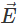as a gradient of a scalar field, which we call as the electric potential. By convention to take the electric field as the negative gradient of potential,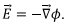The word “potential” reminds us of potential energy. We would like to emphasize that though the two terms are related, potential is not potential energy.

Loosely speaking, the potential is very similar to pressure in a fluid. For instance, if you have a tube containing a liquid, it flows from a region where the pressure is high to a region where the pressure is lower. Similarly, the electric potential is essentially a measure of a “level”. If a positive charge at a point is surrounded by a region of lower potential, it would tend to move towards that region.

Suppose we want to bring a charge q from some reference point A to a point B which is in a region where there exists an electric field E. This electric field could have been produced by other charges that exits in the region. The work that I must do to bring this charge is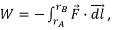where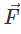is the force that the electric field exerts on the charge and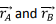are, respectively, the position vectors of the points A and B. The negative sign is because, it is not the force done by the charge but by an external agency, i.e., me. Since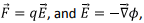the work done by me is W =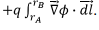In our lecture on vector calculus, we had seen that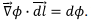Thus we have,

I could choose the reference point A to be the point where the potential is defined to be zero, in which case the work done will be given by the expression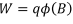This work that is done by an external agency must result in an increase in the potential energy of the system, which consists of our “test charge” q and all other charges in whose field the test charge was made to move. But in this expression for the change in potential energy, there is an explicit reference to the charge q of the test charge. So in some sense, the potential energy that is associated with the test charge q located at the point B is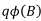and the potential at the point B is nothing but the potential energy associated with a unit charge when it is brought to the point B from wherever the zero of the potential energy might be.

Thus, the potential is not potential energy though there is a deep connection between the two terms. Potential is measured in Joules while the unit of potential is Joule per Coulomb, which is a “Volt”.

Often we deal with curves or surfaces on which the potential remains constant. If a charge is kept on such a surface, it would experience no force because the gradient of potential is zero on such a surface. Such surfaces and curves are known as “Equipotential” surfaces or curves. By definition, they are perpendicular to the electric field lines because no work is done when we move a charge on such a surface.

In the figure, we show the field lines and equipotential surfaces for a single positive charge. We had seen earlier that the field lines are symmetrical and diverge out from the point charge. The equipotential surfaces which are perpendicular to the field lines are, therefore, are family of concentric spheres which their centre at the location of the charge.

We will now calculate the potential in a couple of cases.

The first problem that we consider is the potential due to a charged ring along its axis. We take the charged ring in the xy plane with its centre at the origin. The axis of the ring is the z-axis. Let the linear charge density of the ring be λ. I take an element of the ring at an azimuthal angle φ’, so that the length of the charged element is Rdφ’. We will calculate the potential at a point P, which has the coordinates(0,0,z). We put a prime on the angle φ’ which is an integration variable so as not to confuse with the symbol f that we use for the potential.

The distance of the point P from the charged element is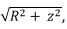which gives the expression for the potential to be given by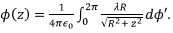Other than the integration variable, everything else in this expression is constant. Since the integration over the angle gives us 2π, we get the potential to be given by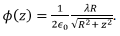The field due to the charged element is along the line joining the element and the point P. But, as I go along the ring, the components of the field perpendicular to the axis cancel by symmetry and the resultant field will be along the z axis. The gradient of the potential is thus just a differentiation with respect to z and we have,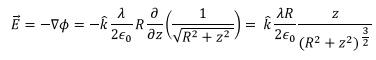which is the final expression for the potential due to a charged ring.

Next, we consider a charged spherical shell with a surface charge density σ. Since it is a shell, the charges are all on the surface of the sphere. Recall that in the spherical polar coordinates, the surface element is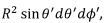where we have put primes to indicate integration variables. We want to calculate the potential at a point which is at a distance r from the centre of the shell. Whichever point you want to calculate the potential, we join that point with the centre and take that to be the z axis. The potential can only depend on the distance z from the centre because of spherical symmetry. (We will denote by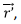the position of an arbitrary charge element, since the charge element is on the surface of the sphere,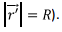As a result the potential at a point at a distance z from the centre is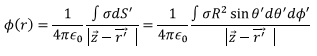By triangle law,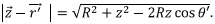The integral has no dependence on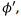so the integral over it gives 2π. To do the θ integral, we make a variable transformation,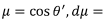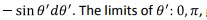gives the limits on µ to be from -1 to +1, which gives, by interchanging the limits to take care of the minus sign in dµ, we have,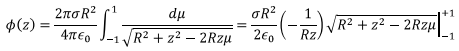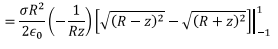The reason for writing it in this form is that we have to be careful and take only the positive square root. For points inside the shell, R>z, and we get,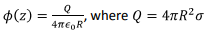is the total charge in the shell. Note that the potential inside is independent of the distance from the centre. For points outside the shell R<z, and we getWe will now replace z with r as the potential depends only on the distance r from the origin. Using a unit step function (theta function), we can combine both these results into a single equation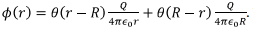The theta function has the property that if its argument is greater than zero it has the value 1; otherwise it is zero (see figure on the screen).

The electric field can be calculated by taking the gradient of the potential. The second term, being constant, does not contribute to the field, only the first term does. Thus the field is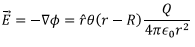So that the field inside is zero. For points outside the sphere, the field is the same as it would be if the entire charge on the shell were concentrated at the centre of the shell.

Let us summarize what we have learnt so far :

1. The potential is spherically symmetric, i.e., it depends only on the distance from the centre.

2. The potential inside the shell is constant. This also follows from Gauss’s law as, the charges being only on the surface, the charge enclosed is zero. So the field would be zero implying that the potential is constant, which we could take it to be zero.

3. If you look at the expression for the potential, you notice that the potential is continuous across the surface because, its value on the surface is the same as its value just outside the surface.

4. The electric field, however, is zero inside and has a non-zero value infinitesimally outside the surface. Thus the electric field is discontinuous across the charged sphere. We will see that this feature is common to all geometries. To see this, let us consider yet another example. Potential due to a charged disk along it axis of symmetry. The problem has cylindrical symmetry.
Take a concentric annulus of radius r’ and width dr’ so that if the charge density is σ, the charge in the annulus is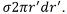All the points on the annulus are at the same distance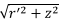from the point P(0,0,z) on the axis. The potential, therefore, is given by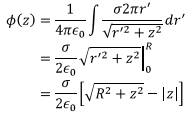where we have written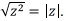Let us take some specific limits. Suppose the point P is far far away from the disk. We can then expand the first term in the above expression by a binomial, and get,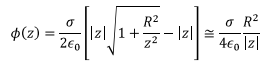If we observe that the total charge on the disk is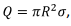the potential becomes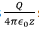so that at large distances, the potential is the same as for a point charge. This is sensible because when the distances are large, the disk, whose dimensions are small compared to the distance appears like a point charge.
More interesting limit however is when the point P is very close to the surface, i.e., R >> z, The expression for potential is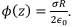Notice that when the point of observation is very close to the disk, the disk appears like an infinite plane. So, the expression for the potential is the same as that due to an infinite charged plane. Things become obvious if you calculate the electric field due to the charged disk. The symmetry being cylindrical, the gradient is just a differentiation with respect to z, and we have,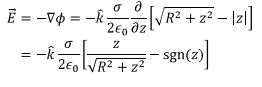The last term requires an explanation. Since the last term of the term to be differentiated is a modulus of z, it is z if z>0, so that the differentiation is +1. On the other hand if z<0, it is –z, so that the differentiation is -1. This is written as “sign” of z and abbreviated as sgn(z). Near the disk, z ≈ 0, the first term vanishes and we are left with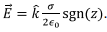Thus above the disk where z>0, the field is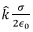whereas for points below the disk, where z<0, the field is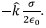Once again you notice that whereas the potential was continuous across the surface, the electric field is discontinuous.
Let me make an observation from the last two examples that I have given. In both cases there is a charged surface and we saw that where as the potential is continuous across the charged surface, the electric field is discontinuous. We will see later that this can be proved quite generally, viz., whenever there is a charged surface, the electric field will have a discontinuity across such a surface.
Let me now take an example where we calculate potential due to a three dimensional charged object. Consider a uniformly charged sphere containing charge Q, so that the charge density is given by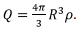This is a problem which we have seen in the past, in connection with Gauss’s law. Recall that according to Gauss’s law, the flux out of a surface (real or imaginary) is given by charge enclosed divided by ∈0. As a result, if I am calculating the field at a distance r from the centre of the sphere, we draw an imaginary surface of radius r concentric with the given sphere. Thus if r>r, the charge enclosed is the total charge Q, and we have,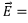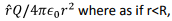the charged enclosed is not the entire charge butbecause that is the fraction of total charge that is included within an imaginary surface of radius r<R In this case, the electric field is given by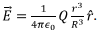I will integrate these expressions to get the potential in these two cases. Considering the case of r>R, the potential V(r) is given by,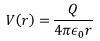Note that we have assumed that the constant of integration is such that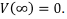Since, we already have a reference point for the zero of potential, we cannot change it midway. And one has to be careful in calculating the potential when rR) and then fo from the surface of the sphere to the point inside the sphere where we need to know the potential. For the second part we take the expression for the electric field for the second part.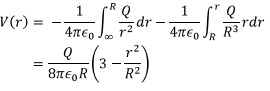Let me now return to this point that we have been making that whenever there is surface charge, the electric field has a discontinuity whereas the potential is continuous. In other words, I am looking at the behavior of electric field and potential at the boundary.

Let us look at a situation like this. I have an arbitrary surface; suppose it is an infinite charged surface. I know how to calculate the electric field both below and above such a charged surface. To do so, let me enclose a part of the surface by a Gaussian surface. I take a rectangular parallelepiped for height ∈ half of which is below the surface and half above it. The flux enclosed by such a parallelepiped is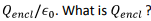If the area enclosed is A, then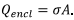Remember that charge is only on the surface. Thus the flux enclosed is given by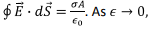the contributions from the sides, other than the upper and the lower surface, vanish, and we have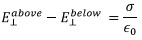Which re-emphasizes that the electric field is discontinuous across the boundary of a charged surface.

To summarize, in this lecture, we have defined electric potential and explained its meaning and its relation with the electric field. We have calculated the potential in a few cases, viz. for a charged ring, a disk and a uniformly charged sphere. We have also seen that the electric field is discontinuous across a charged surface. The last statement is a statement of Electrostatic Boundary Conditions, which we will explore further in the subsequent lecture.

Tutorial :

Obtain an expression for the potential due to a long charged wire (a) by direct integration and (b) using the expression for the electric field. (Note that in this case you cannot take the zero of the potential at infinite distance.)

Solution to Assignment :

(a) Define the origin of the cylindrical coordinate system at the point where the perpendicular from P meets the line charge (taken along the z-axis). Take an element of length dz at z. The potential at P located at a distance ρ from the wire is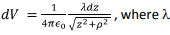is the linear charge density.

The potential at P due to the wire is obtained by integrating this expression from -∞ to +∞. However, it turn out that the integration diverges. The reason is that we have started with an expression where the potential is taken to be zero at infinite distances. This, however, creates a problem because the line charge itself extends to infinity. One has to choose a different reference point for the potential.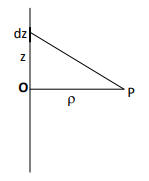Let us take the line to extend from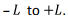The potential due to this wire at P is given by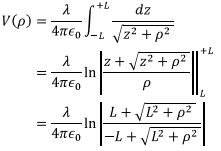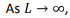the right hand side diverges, as expected. However, the trick is to take the limit carefully and isolate a constant which is infinite. This is done by doing an expansion of the terms in the numerator and denominator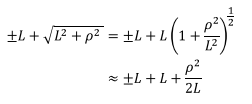Substituting this in the expression for the potential, we get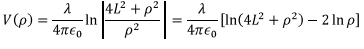The first term inside the bracket goes to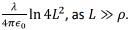This is an infinite constant. If we take the reference point of the potential to be at some distance  p0, the constant would cancel out leaving us with an expression for the potential given by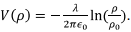(b) We had obtained the electric field due to a line charge using Gauss’s law. The field is given by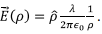Because of cylindrical symmetry, the gradient is just a differentiation with respect to ρ. Thus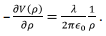Integrating, we get,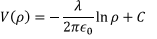Once again, we see that we cannot take the reference point at infinite distances. Taking the reference point at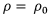will give us the same expression as obtained in part (a).

Self Assessment Quiz

1. A charge + Q is uniformly distributed over an annulus with an inner radius R and outer radius 2R. find the potential at a distance z from the annulus along its axis.

2. A positive charge +q is released from rest from the centre of the annulus of Problem 1 above. What is the final velocity attained by this mass.

3. An infinite charged plane having a surface charge density σ is perpendicular to the z-axis at z=0. Obtain an expression for the electric potential and sketch the potential function.

4. A sphere of radius R has a charge p(r) = A/r density inside the sphere where r is the distance from the origin. Find the electric field and obtain an expression for the potential from the field.

Solutions to Self Assessment Quiz

The charge density in the annulus is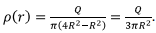Take a ring of radius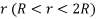of width dr. The charge in the ring is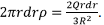The potential due to this annulus at z is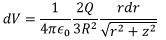Integrating over the annulus, we have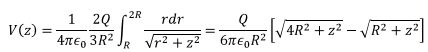2. If a charge is released from the centre of the annulus (z=0) its potential energy is given by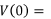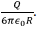Note that the potential energy at infinite distance is zero (take z>>R in the expression for V(z)). Thus at far distances, all the initial potential energy becomes the kinetic energy of the particle. Equating this to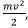the velocity can be found.

3. The electric field is given by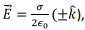where the upper sign is to be taken for z>0 and the lower sign for z<0 (there is a discontinuity of the electric field at a charged surface). Thus above the plane, the potential is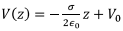and below the plane, it is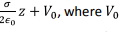is an integration constant. Note that the potential is continuous at z=0, the boundary. A sketch of the potential is as follows :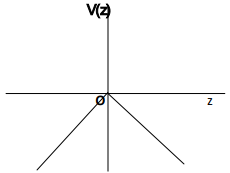4. Total charge is obtained by integrating the charge density over the volume,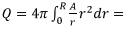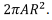The amount of charge enclosed inside a spherical surface of radius r is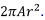Using Gauss’s law, the electric field inside the sphere is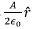and outside the sphere it is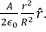Because of spherical symmetry, the gradient is simply a differentiation with respect to r. Inside the sphere,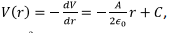where C is a constant Outside the sphere, the potential is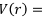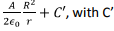being constant. The potential being continuous at the surface r = R, the constants must be chosen so that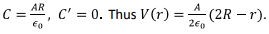The document Potential of a Charge Distribution - Notes | Study Electromagnetic Fields Theory (EMFT) - Electrical Engineering (EE) is a part of the Electrical Engineering (EE) Course Electromagnetic Fields Theory (EMFT).
All you need of Electrical Engineering (EE) at this link: Electrical Engineering (EE)

## Electromagnetic Fields Theory (EMFT)

11 videos|46 docs|62 tests
 Use Code STAYHOME200 and get INR 200 additional OFF

## Electromagnetic Fields Theory (EMFT)

11 videos|46 docs|62 tests

Track your progress, build streaks, highlight & save important lessons and more!

,

,

,

,

,

,

,

,

,

,

,

,

,

,

,

,

,

,

,

,

,

;